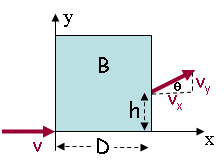# Motion In A Magnetic Field 2

Baumer8993

## Homework Statement

A proton (q = 1.6 X 10-19 C, m = 1.67 X 10-27 kg) moving with constant velocity enters a region containing a constant magnetic field that is directed along the z-axis at (x,y) = (0,0) as shown. The magnetic field extends for a distance D = 0.79 m in the x-direction. The proton leaves the field having a velocity vector (vx, vy) = (4.4 X 105 m/s, 2.2 X 105 m/s).

What is the radius of curvature of the proton while it is in the region of the magnetic field?## Homework Equations

V*M = Q*B*R

V-Velocity
M-Mass Of Particle
Q-Charge Of Particle
B-Magnetic Field

## The Attempt at a Solution

I got what the velocity entering was since the particle does not speed up from the magnetic field. I know the particle moves in a circular path. I am just stuck on how to find the radius of the circle. From there everything else will fall into place.

Homework Helper
Gold Member
1 I know the particle moves in a circular path. I am just stuck on how to find the radius of the circle. From there everything else will fall into place.

You know that the center of the circle must be on the y-axis (if it wasn't then it wouldn't be perpendicular to the initial V.

You also have an idea of another slanted line that starts at the center of the circle and ends at the place where the proton exits, and that line is perpendicular to the final trajectory of the proton. Try to draw that wedge out. It will look like a section of pie or pizza. The bottom will be a [STRIKE]curved line[/STRIKE] [arc] indicating the path of the proton.

Now, you already have θ defined in your diagram, and you know that tanθ = Vy/Vx.

Now try to find/draw a similar triangle (in the wedge somewhere) that includes the circle's radius, θ, and D.

Last edited:
•1 person
Baumer8993
Can you explain the wedge part a little more? I am a little confused by what you mean.

Homework Helper
Gold Member
I mean the center of the circle will be above the x-axis, on the y-axis somewhere. You don't know exactly how far up the y-axis it is yet, but don't worry, you can find that out later.

On a figure, draw a point where you think the center of the circle might be (it will be somewhere up on the y-axis).

The length of the that line is the radius of the circle. Now draw another line, with the same length that starts at the center-point of the circle and ends at the location where the proton exits the magnetic field.

Connecting the bottom of the two, equidistant lines is the path of the proton, which makes an arc. All together, the two lines and the arc form a "wedge." It's in the shape of a piece of pie or a piece of pizza.

How does the geometry of this wedge relate to θ?

By the way, on a different note, there is yet another triangle you can form. How does θ relate to h and D [and the radius of the circle]? [Edit: On second thought, you probably don't need to calculate this if you don't want to. I don't think it's necessary to solve the problem.]

Last edited:
•1 person
Baumer8993
Ahh that makes sense! Thank you very much!

Baumer8993
There we go! That let me get the entire problem done! Thanks again!